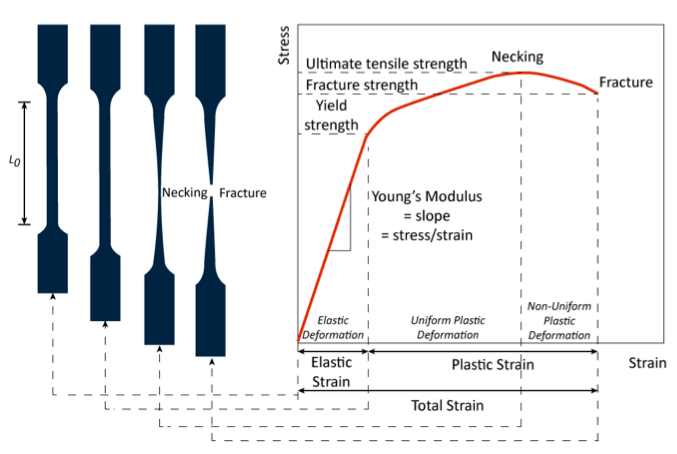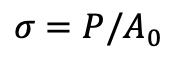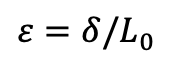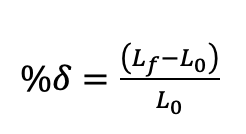# What is Elongation?

Mechanical properties are essential in describing the behaviour of a material when a load is applied. Many tests, such as tension, compression, impact, bending, shear, torsion, and hardness tests, are developed in order to observe the response of a material to an applied external force. These properties are important in order for engineering designers to select appropriate materials for different applications.

## What is the elongation of a material?

Elongation is a measure of deformation that occurs before a material eventually breaks when subjected to a tensile load. As the latter is applied, an increase in length and a uniform reduction in cross-sectional area take place, while the material maintains a constant volume. Elongation due to expansion can also occur when a material undergoes an increase in temperature, or if both an axial force and a high temperature act simultaneously upon a material.

## How is elongation measured?

Measuring material elongation due to axial force is usually carried out by a standard tensile strength test. A strip or rod with a certain length and a uniform cross-sectional area, fixed at one end, is subjected to a tensile load along the specimen’s axis. Gauge marks are scribed on the specimen as it is placed in the grips of the testing machine to define the gauge length. The axial load is increased incrementally and elongation is observed until the material mechanically breaks or fractures . This test is usually normalised, realised at constant load velocity, and is destructive. The gauge length or calibration length must be long enough in order to ensure that the tensile stress is transmitted uniformly.A typical progression of a tensile test begins with the specimen situated in the absence of a load. At the initial application of the load, uniform elongation and reduction of the cross-sectional area can be observed. This continues until a maximum load is reached. Thereupon, necking occurs, causing the following deformation to become non-uniform and take place merely at the neck. The local true stress continues to increase as the neck area decreases until fracture is reached.

The formula for elongation at any length L during tensile testing is:where,

δ = elongation, (in or mm)

L0 = initial gauge length between marks, (in or mm)

L = length between marks at any point during uniform elongation, (in or mm)

During a tensile test, readings of the applied load and the elongation of the gauge length are taken simultaneously. This data can be plotted on a graph called the stress-strain curve. The stress-strain curve is essential in understanding the material properties as it shows the basic relationship between the engineering stress and the engineering strain .

The formula for engineering stress, showing an influence by the applied load over the original cross-sectional area, is:where,

σ = engineering stress, (lb/sq. in or MPa)

P = applied load, (lb or N)

A0 = original cross-sectional area, (sq. in or sq. mm)

The engineering strain, on the other hand, is measured as the ratio of the elongation to the gauge length:where,

ε = engineering strain, (unitless)

δ = elongation at any point during uniform elongation stage, (in or mm)

L0 = initial gauge length between marks (in or mm) 

## What is percent elongation?

Suppose we have a rod that has undergone a tensile strength test. Using an extensometer, we can measure the original gauge length Lo and a final gauge length Lf after necking and fracture. The ratio of the difference in the final length and original length to the original length itself is known as percent elongation (%δ).where,

%δ = percent elongation, (%)

Lf = final specimen length, (in or mm)

Lo = original specimen length, (in or mm)

This measurement represents a material property called ductility .

## Why calculate material elongation?

Conducting a tensile test to determine the elongation of different materials is important in order to evaluate their possible uses. For example, materials with high elongation – i.e. good ductility – are critical in components that require energy absorption by plastic deformation, such as crash barriers and bumpers.

For fabrication processes, knowing this property is essential for implementing quality control metrics.

For material and manufacturing engineers, such properties are studied in order to assess material weakness and limitations for improvement and exploration of alternatives .